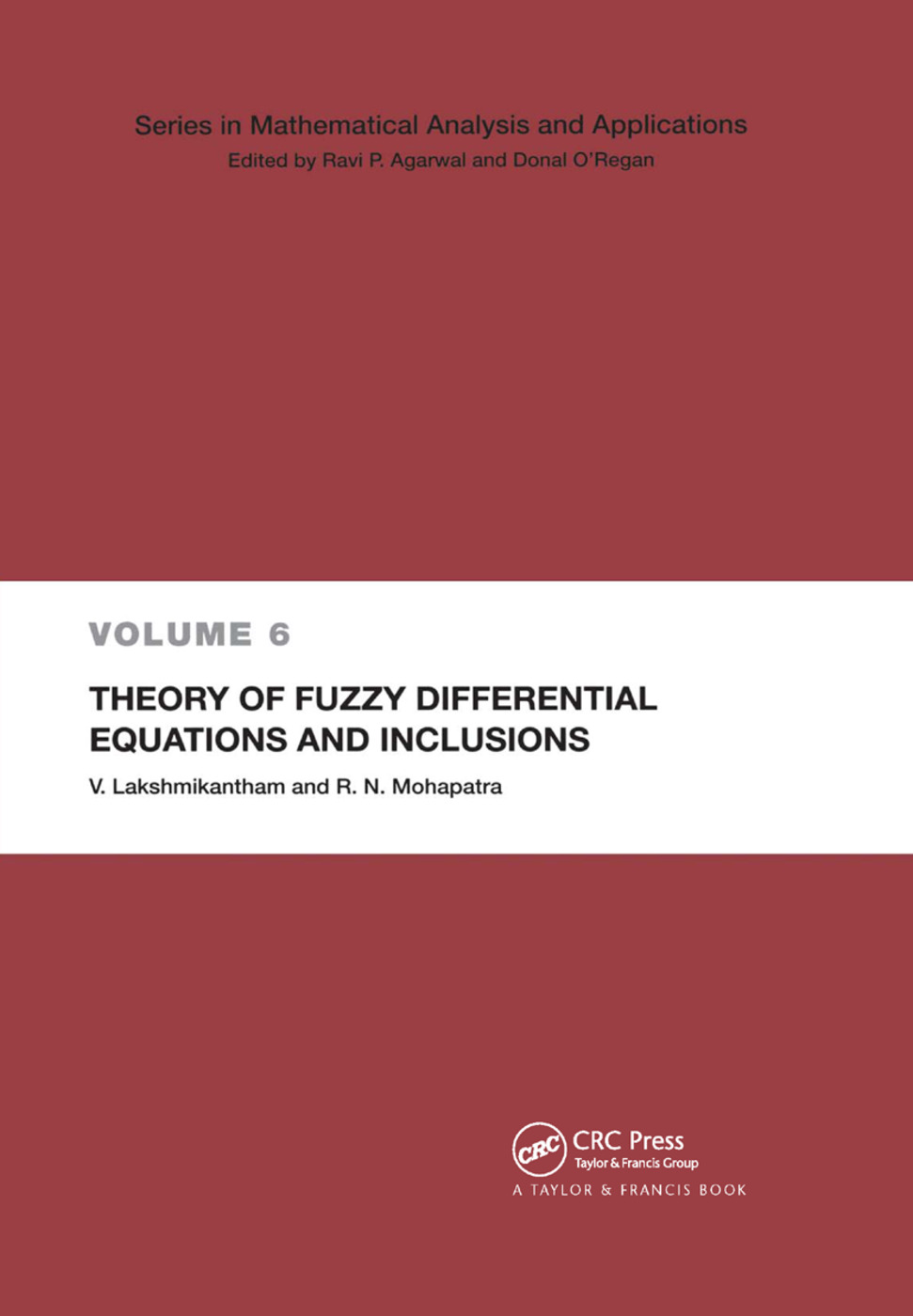# Theory of Fuzzy Differential Equations and Inclusions

## 1st Edition

CRC Press

190 pages

Paperback: 9780367395322
pub: 2019-09-11
SAVE ~\$14.99
\$74.95
\$59.96
x
Hardback: 9780415300735
pub: 2003-03-20
SAVE ~\$29.00
\$145.00
\$116.00
x

FREE Standard Shipping!

### Description

Fuzzy differential functions are applicable to real-world problems in engineering, computer science, and social science. That relevance makes for rapid development of new ideas and theories. This volume is a timely introduction to the subject that describes the current state of the theory of fuzzy differential equations and inclusions and provides a systematic account of recent developments. The chapters are presented in a clear and logical way and include the preliminary material for fuzzy set theory; a description of calculus for fuzzy functions, an investigation of the basic theory of fuzzy differential equations, and an introduction to fuzzy differential inclusions.

Fuzzy Sets. The Hausdirfi Metric. Support Functions. The Space E^Tn. The Metric Space. Calculations of Fuzzy Functions. Convergence of Fuzzy Sets. Measurability. Integrability. Differentiability. Fundamental Theory. Initial Value Problem. Existence. Comparison Theorems. Convergence of Successive Approximations. Continuous Dependence. Global Existence. Approximate Solutions. Stability Criteria. Lyapunov-like Functions. Nonuniform Stability Criteria. Criteria for Boundedness. Fuzzy Differential Systems. The Method of Vector Lyapunov Functions. Linear Variation of Parameters Formula. Fuzzy Difference Equations. Impulsive Fuzzy Differential Equations. Fuzzy DEs with Delay. Hybrid Fuzzy Differential Equations. Fixed Points of Fuzzy Mappings. Boundary Value Problem. Fuzzy Equations of Volterra Type. A New Concept of Stability. Fuzzy Differential Inclusions. Formulation of FDIs. Differential Inclusions. Variation of Constants Formula. Fuzzy Volterra Integral Equations. Bibliography.

Lakshmikantham, V.; Mohapatra, Ram N.